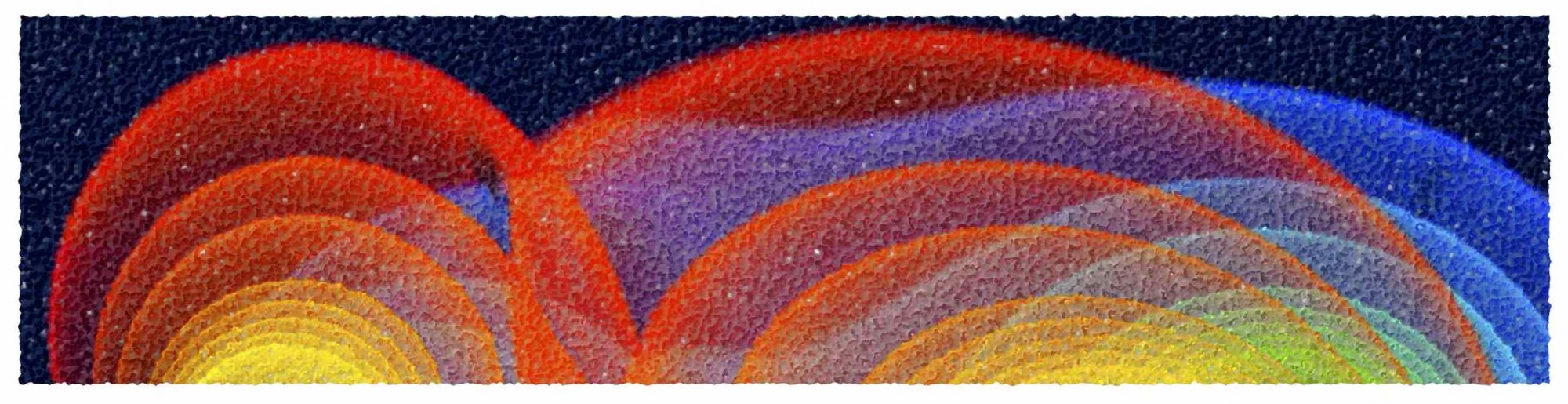# Numerical Methods for Physics

The overview of the lecture can be found here.

The solutions will appear here once they are ready for publication:

• Root Finding
• Linear Algebra
• Interpolation and Extrapolation of functions
• Integration of Functions
• Random Numbers and Montercarlo Techniques
• Solution of Ordinary Differential Equations
• Solution of Partial Differential Equations
• Hyperbolic Equations
• Parabolic Equations
• Elliptic Equations
• Fourier Transforms
• Data Analysis Techniques